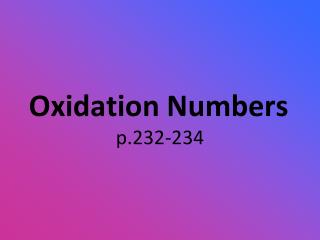DownloadDownload PresentationOxidation Numbers

# Oxidation Numbers

Télécharger la présentation## Oxidation Numbers

- - - - - - - - - - - - - - - - - - - - - - - - - - - E N D - - - - - - - - - - - - - - - - - - - - - - - - - - -
##### Presentation Transcript

1. Oxidation Numbers p.232-234

2. Assigning Oxidation Numbers • Oxidation Number: positive or negative number assigned to an atom according to a set of rules

3. Rules for Assigning Oxidation Numbers • The oxidation number of any free element is 0 - Na or O2 • The oxidation number of a monatomic ion is equal to the charge on the ion. Some atoms have several possible oxidation numbers. - Iron can be Fe2+ or Fe3+

4. Rules for Assigning Oxidation Numbers • The oxidation number of each hydrogen atom in most of its compounds is +1, EXCEPT in metal hydrides (LiH) where it is -1. • The oxidation number of oxygenIN A COMPOUND is -2, EXCEPT in peroxides (H2O2) where it is -1.

5. Rules for Assigning Oxidation Numbers • The sum of the oxidation numbers of ALL THE ATOMS in a particle must equal the apparent charge of that particle. SO42-, S2O32-, Na2SO4 • In compounds, the elements of Group 1, 2, and aluminum have positive oxidation #’s of +1, +2, and +3 respectively. AlBr3, CaO

6. Now you try some! • Find the oxidation numbers of iodine in HIO4, HIO3, HIO, HI • Section Review p. 235 #1 a-i

7. Oxidation-number Changes in Chemical Reactions • If the oxidation number increases for an atom • Oxidation has occurred • If the oxidation number decreases for an atom • Reduction has occurred 2AgNO3(aq) + Cu(s)  Cu(NO3)2(aq) + 2Ag(s) • What is being oxidized? What is being reduced? +1 +5 –2 0 +2 +5 –2 0

8. Now you try some! • Use the changes in oxidation numbers to identify which atoms are oxidized, and which atoms are reduced: 2H2 (g) + O2 (g)  2H2O (l) 2KNO3 (s)  2KNO2 (s) + O2 (g) NH4NO3 (s)  N2 (g) + 2H2O (g) (HINT: consider each N separately) PbO2 (aq) + 4HI (aq)  I2 (aq) + PbI2 (s) + 2H2O (l)